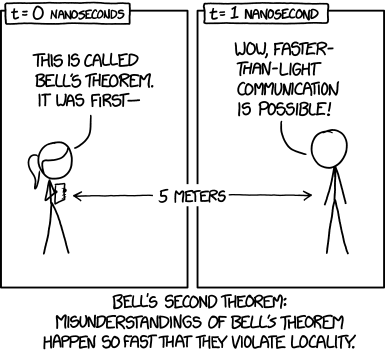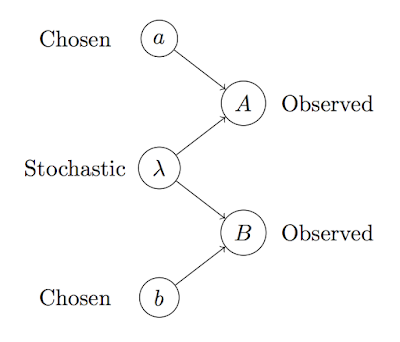## Thursday, October 22, 2015

### Bell: "Bertlmann's Socks and the Nature of Reality" (1980)

There seems to be a lot of mystery surrounding Bell's theorems. Scholarpedia has a whole section devoted to "controversy and common misunderstandings" surrounding the argument, and a recent xkcd comic took up the topic (without mentioning any specific misunderstanding).Source: xkcd.com/1591

I've also been told in person that I understood the theorem wrong. So it seems about time for some studying.

This time, rather than pick up Bell's original article, I read this more popular account of the argument, which covers more or less the same ground. If I understand it correctly, it's actually simpler than what I first thought, although my hazy understanding of the physics stood in the way of extracting the purely statistical part of the argument.

### Background

Here's what I take to be the issue: We have a certain experiment in which two binary observables, $A$ and $B$, follow conditional distributions that depend on two control variables, $a$ and $b$:\begin{eqnarray}
a &\longrightarrow& A \\
b &\longrightarrow& B
\end{eqnarray}Although the experiment is designed to prevent statistical dependencies between $A$ and $B$, we still observe a marked correlation between them for many settings of $a$ and $b$. This has to be explained somehow, either by postulating
• an unobserved common cause: $\lambda\rightarrow A,B$;
• an observed common effect: $A,B \rightarrow \gamma$ (i.e., a sampling bias);
• or a direct causal link: $A \leftrightarrow B$.
The purpose of Bell's paper is to rule out the most plausible and attractive of these three options, the hidden common cause. This explanation is ruled out by showing that a certain measure of dependence would exceed a logically necessary bound under this type of explanation.

### Measurable Consequences

The measure in question is the following:
\begin{eqnarray}
C(a,b) &=& +P(A=1,B=1\,|\,a,b) \\
&   & +P(A=0,B=0\,|\,a,b) \\
&  &  -P(A=1,B=0\,|\,a,b) \\
&  &  -P(A=0,B=1\,|\,a,b).
\end{eqnarray}This statistic is related to the correlation between $A$ and $B$ but different due to the absence of marginal probabilities $P(A)$ and $P(B)$. It evaluates to $+1$ if and only if the two are perfectly correlated, and $-1$ if and only if they are perfectly anti-correlated.Contours of $C(a,b)$ when $A$ and $B$ are independent with $x=P(A)$ and $y=P(B)$.

In a certain type of experiment, where $a$ and $b$ are angles of two magnets used to reveal something about the spin of a particle, quantum mechanics predicts that
$$C(a,b) \;=\; -\cos(a-b).$$When the control variables only differ little, $A$ and $B$ are thus strongly anti-correlated, but when the control variables are on opposite sides of the unit circle, $A$ and $B$ are closely correlated. This is a prediction based on physical considerations.

### Bounds on Joint Correlations

However, let's stick with the pure statistics a bit longer. Suppose again $A$ depends only on $a$, and $B$ depends only on $b$, possibly given some fixed, shared background information which is independent of the control variables.The statistical situation when the background information is held constant.

Then $C(a,b)$ can be expanded to
\begin{eqnarray}
C(a,b) &=& +P(A=1\,|\,a) \, P(B=1\,|\,b) \\
&   & +P(A=0\,|\,a) \, P(B=0\,|\,b) \\
&   & - P(A=1\,|\,a) \, P(B=0\,|\,b) \\
&   & - P(A=0\,|\,a) \, P(B=1\,|\,b) \\
&=& [P(A=1\,|\,a) - P(A=0\,|\,a)] \times [P(B=1\,|\,b) - P(B=0\,|\,b)],
\end{eqnarray}that is, the product of two statistics which measure how stochastic the variables $A$ and $B$ are given the control parameter settings. Using obvious abbreviations,
$$C(a,b) \; = \; (A_1 - A_0) (B_1 - B_0),$$and thus
\begin{eqnarray}
C(a,b) + C(a,b^\prime) &=&
(A_1 - A_0) (B_1 - B_0 + B_1^\prime - B_0^\prime)
& \leq & (B_1 - B_0 + B_1^\prime - B_0^\prime); \\
C(a^\prime,b) - C(a^\prime,b^\prime) &=& (A_1^\prime - A_0^\prime) (B_1 - B_0 - B_1^\prime + B_0^\prime)
& \leq & (B_1 - B_0 - B_1^\prime + B_0^\prime).
\end{eqnarray}It follows that
$$C(a,b) + C(a,b^\prime) + C(a^\prime,b) - C(a^\prime,b^\prime) \;\leq\; 2(B_1 - B_0) \;\leq\; 2.$$Since $(B_1 - B_0)\geq-1$, a similar derivation shows that
$$| C(a,b) + C(a,b^\prime) + C(a^\prime,b) - C(a^\prime,b^\prime) | \;\leq\; 2|B_1 - B_0| \;\leq\; 2.$$In fact, all 16 variants of this inequality, with the signs alternating in all possible ways, can be derived using the same idea.

### Violations of Those Bounds

But now look again at
$$C(a,b) \;=\; -\cos(a-b).$$We then have, for $(a,b,a^\prime,b^\prime)=(0,\pi/4,\pi/2,-\pi/4)$,
$$\left| C\left(0, \frac{\pi}{4}\right) + C\left(0, -\frac{\pi}{4}\right) + C\left(\frac{\pi}{4}, \frac{\pi}{4}\right) - C\left(\frac{\pi}{4}, -\frac{\pi}{4}\right) \right| \;=\; -2\sqrt{2},$$which is indeed outside the interval $[-2,2]$. $C$ can thus not be of the predicted functional form and at the same time satisfy the bound on the correlation statistics. Something's gotta give.

### Introducing Hidden Variables

This entire derivation relied on $A$ and $B$ depending on nothing other than their own private control variables, $a$ and $b$.

However, suppose that a clever physicist proposes to explain the dependence between $A$ and $B$ by postulating some unobserved hidden cause influencing them both. There is then some stochastic variable $\lambda$ which is independent of the control variables, yet causally influences both $A$ and $B$.The statistical situation when the background information varies stochastically.

However, even if this is the case, we can go through the entire derivation above, adding "given $\lambda$" to every single step of the process. As long as we condition on a fixed value of lambda, each of the steps still hold. But since the inequality thus is valid for every single value of $\lambda$, it is also valid in expectation, and we can thus integrate $\lambda$ out; the result is that even under such a "hidden variable theory," the inequality still holds.

Hence, the statistical dependency cannot be explained by a shared cause alone, since the functional form of the probability densities for $A$ given $a$ and $B$ given $b$ are of a wrong form. We will therefore need to either postulate direct causality between $A$ and $B$ or an observed downstream variable (sampling bias) instead.

Note that the only thing we really need to prove this result is the assumption that the probability $P(A,B \, | \, a,b,\lambda)$ factors into the product $P(A \, | \, a,b,\lambda)\, P(B \, | \, a,b,\lambda)$. This corresponds to the assumption that there is no direct causal connection between $A$ and $B$.